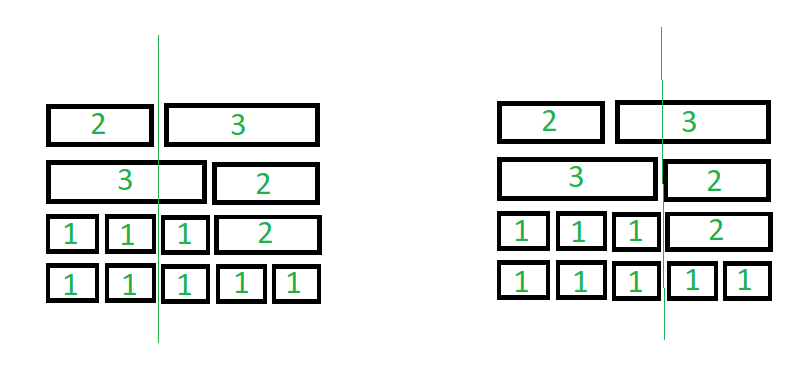Related Articles

# Minimize count of tiles cut in a single strike

• Last Updated : 28 Jul, 2021

Given a 2D array representing width and level of tiles of equal length, with each level of same total width, the task is to find the minimum number of tiles that can be cut by a single vertical strike of sword passing through all the levels of the tiles.

Examples:

Input: tilesStack[][] = { { 2, 3 }, { 3, 2 }, { 1, 1, 1, 2 }, { 1, 1, 1, 1, 1 } }
Output:
Explanation:
The minimum number of tiles that can be cut is equal to 1Input: tilesStack[][] = { { 1, 1 }, { 1, 1 } }
Output: 0

Approach: The idea is to find the maximum number of gaps so that if a vertical line drawn passing through those points, then it will cut the minimum number of tiles. Follow the steps below to solve the problem:

• Traverse each row of the 2D array tilesStack[][]. For every ith row, Map its prefix sum, which represents the distance of gaps starting from the leftmost tile and store the frequency of prefix sum of each row using Map.
• Find the largest frequency of prefix sum (of distance of gaps), say X.
• Finally, print the value of (length(tilesStack) – X), which represent the minimum number of tiles that gets cut by drawing a vertical line.

Below is the implementation of this approach.

## C++

 `// C++ program for the above approach``#include``using` `namespace` `std;` `// Function to count the minimum number``// of tiles that gets cut by a single``// vertical strike of a sword``void` `cutTiles(vector> tilesStack)``{` `  ``// Map prefix sum of each row``  ``// of tilesStack``  ``map<``int``, ``int``> gaps;` `  ``// Traverse each row of the tiles``  ``for``(vector<``int``> tiles:tilesStack)``  ``{` `    ``// Stores distance of gap from``    ``// left of each row``    ``int` `totWidth = 0;` `    ``// Excluding the last gap as it will``    ``// be the edge of the level``    ``for``(``int` `i = 0; i < tiles.size() - 1; i++)``    ``{` `      ``// Update totWidth``      ``totWidth += tiles[i];` `      ``// If gap is already found at``      ``// this points in previous levels``      ``gaps[totWidth]++;``    ``}``  ``}` `  ``// Stores maximum number of ``  ``// gap from left``  ``int` `X = 0;``  ``for``(``auto` `x : gaps)``  ``{``    ``X = max(x.second, X);``  ``}``  ``cout << tilesStack.size() - X;``}` `// Driver code``int` `main()``{``  ``vector> tilesStack(4);``  ``tilesStack.push_back(2);``  ``tilesStack.push_back(3);``  ``tilesStack.push_back(3);``  ``tilesStack.push_back(2);``  ``tilesStack.push_back(1);``  ``tilesStack.push_back(1);``  ``tilesStack.push_back(1);``  ``tilesStack.push_back(2);``  ``tilesStack.push_back(1);``  ``tilesStack.push_back(1);``  ``tilesStack.push_back(1);``  ``tilesStack.push_back(1);``  ``tilesStack.push_back(1);` `  ``// Function Call``  ``cutTiles(tilesStack);``}` `// This code is contributed by rutvik_56.`

## Java

 `// Java program for the above approach``import` `java.util.*;``public` `class` `GFG``{` `  ``// Function to count the minimum number``  ``// of tiles that gets cut by a single``  ``// vertical strike of a sword``  ``static` `void` `cutTiles(Vector> tilesStack)``  ``{` `    ``// Map prefix sum of each row``    ``// of tilesStack``    ``HashMap gaps = ``new` `HashMap<>();` `    ``// Traverse each row of the tiles``    ``for``(Vector tiles : tilesStack)``    ``{` `      ``// Stores distance of gap from``      ``// left of each row``      ``int` `totWidth = ``0``;` `      ``// Excluding the last gap as it will``      ``// be the edge of the level``      ``for``(``int` `i = ``0``; i < tiles.size() - ``1``; i++)``      ``{` `        ``// Update totWidth``        ``totWidth += tiles.get(i);` `        ``// If gap is already found at``        ``// this points in previous levels``        ``if``(gaps.containsKey(totWidth))``        ``{``          ``gaps.put(totWidth, gaps.get(totWidth) + ``1``);``        ``}``        ``else``{``          ``gaps.put(totWidth, ``1``);``        ``}``      ``}``    ``}` `    ``// Stores maximum number of ``    ``// gap from left``    ``int` `X = ``0``;``    ``for` `(Map.Entry Key : gaps.entrySet())``    ``{``      ``X = Math.max((``int``)Key.getValue(),X);``    ``}   ``    ``System.out.print(tilesStack.size() - X);``  ``}` `  ``// Driver code``  ``public` `static` `void` `main(String[] args)``  ``{``    ``Vector> tilesStack = ``new` `Vector>();``    ``tilesStack.add(``new` `Vector());``    ``tilesStack.get(``0``).add(``2``);``    ``tilesStack.get(``0``).add(``3``);``    ``tilesStack.add(``new` `Vector());``    ``tilesStack.get(``1``).add(``3``);``    ``tilesStack.get(``1``).add(``2``);``    ``tilesStack.add(``new` `Vector());``    ``tilesStack.get(``2``).add(``1``);``    ``tilesStack.get(``2``).add(``1``);``    ``tilesStack.get(``2``).add(``1``);``    ``tilesStack.get(``2``).add(``2``);``    ``tilesStack.add(``new` `Vector());``    ``tilesStack.get(``3``).add(``1``);``    ``tilesStack.get(``3``).add(``1``);``    ``tilesStack.get(``3``).add(``1``);``    ``tilesStack.get(``3``).add(``1``);``    ``tilesStack.get(``3``).add(``1``);` `    ``// Function Call``    ``cutTiles(tilesStack);``  ``}``}` `// This code is contributed by divyeshrabadiya07.`

## Python

 `# Python3 program for the above approach` `# Function to count the minimum number``# of tiles that gets cut by a single``# vertical strike of a sword``def` `cutTiles(tilesStack):``    ` `    ` `    ``# Map prefix sum of each row``    ``# of tilesStack``    ``gaps ``=` `{}` `    ``# Handling the case when``    ``# map will be empty``    ``gaps[``-``1``] ``=` `0` `    ``# Traverse each row of the tiles``    ``for` `tiles ``in` `tilesStack:` `        ``# Stores distance of gap from``        ``# left of each row``        ``totWidth ``=` `0` `        ``# Excluding the last gap as it will``        ``# be the edge of the level``        ``for` `tile ``in` `tiles[:``-``1``]:` `            ``# Update totWidth``            ``totWidth ``+``=` `tile`  `            ``# If gap is already found at``            ``# this points in previous levels``            ``if` `totWidth ``in` `gaps:``                ``gaps[totWidth] ``+``=` `1``            ``else``:``                ``gaps[totWidth] ``=` `1`  `    ``# Stores maximum number of``    ``# gap from left``    ``X ``=` `max``(``list``(gaps.values()))``    ` `    ``print``(``len``(tilesStack) ``-` `X)`  `# Driver Code``if` `__name__ ``=``=` `'__main__'``:``    ` `    ``tilesStack ``=` `[[``2``, ``3``], [``3``, ``2``], [``1``, ``1``, ``1``, ``2``],``                              ``[``1``, ``1``, ``1``, ``1``, ``1``]]` `    ``# Function Call``    ``cutTiles(tilesStack)`

## C#

 `// C# program for the above approach``using` `System;``using` `System.Collections.Generic;``class` `GFG {``    ` `    ``// Function to count the minimum number``    ``// of tiles that gets cut by a single``    ``// vertical strike of a sword``    ``static` `void` `cutTiles(List> tilesStack)``    ``{``        ``// Map prefix sum of each row``        ``// of tilesStack``        ``Dictionary<``int``, ``int``> gaps = ``new` `Dictionary<``int``, ``int``>();``      ` `        ``// Traverse each row of the tiles``        ``foreach``(List<``int``> tiles ``in` `tilesStack)``        ``{``            ``// Stores distance of gap from``            ``// left of each row``            ``int` `totWidth = 0;``      ` `            ``// Excluding the last gap as it will``            ``// be the edge of the level``            ``for``(``int` `i = 0; i < tiles.Count - 1; i++)``            ``{``                ``// Update totWidth``                ``totWidth += tiles[i];``                ` `                ``// If gap is already found at``                ``// this points in previous levels``                ``if``(gaps.ContainsKey(totWidth))``                ``{``                    ``gaps[totWidth] += 1;``                ``}``                ``else``{``                    ``gaps[totWidth] = 1;``                ``}``            ``}``        ``}``      ` `        ``// Stores maximum number of ``        ``// gap from left``        ``int` `X = 0;``        ``foreach``(KeyValuePair<``int``, ``int``> Key ``in` `gaps)``        ``{``            ``X = Math.Max(Key.Value,X);``        ``}``          ` `        ``Console.WriteLine(tilesStack.Count - X);``    ``}``    ` `  ``static` `void` `Main() {``    ``List> tilesStack = ``new` `List>();``    ``tilesStack.Add(``new` `List<``int``>());``    ``tilesStack.Add(2);``    ``tilesStack.Add(3);``    ``tilesStack.Add(``new` `List<``int``>());``    ``tilesStack.Add(3);``    ``tilesStack.Add(2);``    ``tilesStack.Add(``new` `List<``int``>());``    ``tilesStack.Add(1);``    ``tilesStack.Add(1);``    ``tilesStack.Add(1);``    ``tilesStack.Add(2);``    ``tilesStack.Add(``new` `List<``int``>());``    ``tilesStack.Add(1);``    ``tilesStack.Add(1);``    ``tilesStack.Add(1);``    ``tilesStack.Add(1);``    ``tilesStack.Add(1);``  ` `    ``// Function Call``    ``cutTiles(tilesStack);``  ``}``}` `// This code is contributed by divyeshrbadiya07.`

## Javascript

 ``
Output:
`1`

Time Complexity: O(N * M), where N is total count of levels and M is width of each level
Auxiliary Space: O(M)

Attention reader! Don’t stop learning now. Get hold of all the important mathematical concepts for competitive programming with the Essential Maths for CP Course at a student-friendly price. To complete your preparation from learning a language to DS Algo and many more,  please refer Complete Interview Preparation Course.

My Personal Notes arrow_drop_up